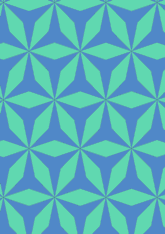# Derived from 3**6 with digons addeddata145/D666

## Geometry

• The symmetry group of the tiling is *632 (p6m).
• All the internal angles of the constituent polygons are a multiple of 8.57 (π/21)°.
• Contains one regular two-pointed star polygon with vertex angle of 34.28 (4π/21)°.
• Contains one regular three-pointed star polygon with vertex angle of 25.71 (π/7)°.
• The tiling satisfies the interlace condition and has no finite interlace and one infinite interlace with non-straight cross-overs.
• The tiling is edge-to-edge.
• As drawn, contains about 142 polygons.

## References

Publications referenced:
1. Uniform Star Polygons with Digons of B A Wichmann. The World of Patterns, World Scientific, 2001. ISBN 981024619. [wich2] {Only new patterns ascribed to this.}

v53